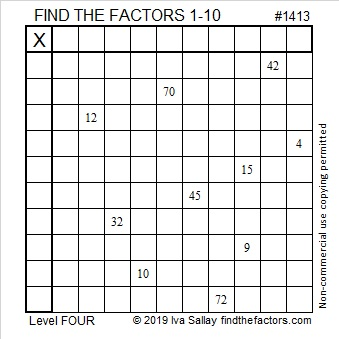# 1413 and Level 4

You find a rhythm as you solve this level 4 puzzle. The logic is quite easy for most of it, but there is at least one place that will require you to think things through before proceeding.Print the puzzles or type the solution in this excel file: 10 Factors 1410-1418

Now I’ll share a few facts about the puzzle number, 1413:

• 1413 is a composite number.
• Prime factorization: 1413 = 3 × 3 × 157, which can be written 1413 = 3² × 157.
• 1413 has at least one exponent greater than 1 in its prime factorization so √1413 can be simplified. Taking the factor pair from the factor pair table below with the largest square number factor, we get √1413 = (√9)(√157) = 3√157.
• The exponents in the prime factorization are 2 and 1. Adding one to each exponent and multiplying we get (2 + 1)(1 + 1) = 3 × 2 = 6. Therefore 1413 has exactly 6 factors.
• The factors of 1413 are outlined with their factor pair partners in the graphic below.1413 is the sum of two squares:
33² + 18² = 1413

That means that 1413 is the hypotenuse of a Pythagorean triple:
765-1188-1413 calculated from 33² – 18², 2(33)(18), 33² + 18².
It is also 9 times (85-132-157)

This site uses Akismet to reduce spam. Learn how your comment data is processed.